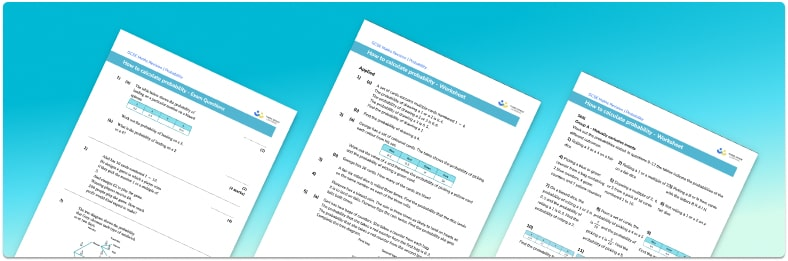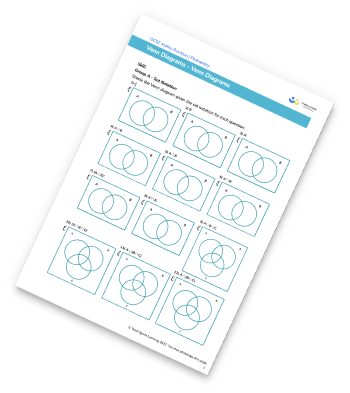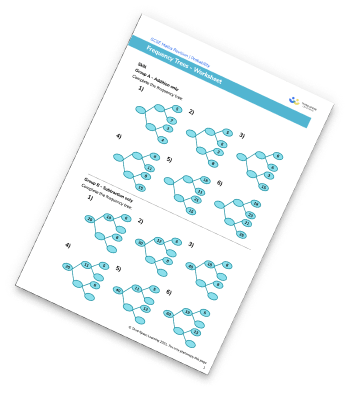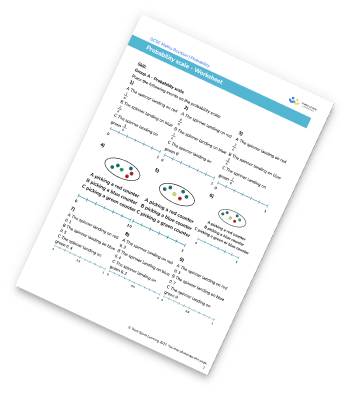# Probability Worksheet• This field is for validation purposes and should be left unchanged.

You can unsubscribe at any time (each email we send will contain an easy way to unsubscribe). To find out more about how we use your data, see our privacy policy.

• Section 1 of the probability worksheet contains 36 skills-based probability questions, in 3 groups to support differentiation
• Section 2 contains 4 applied probability questions with a mix of worded problems and deeper problem solving questions
• Section 3 contains 3 foundation and higher level GCSE exam style probability questions
• Answers and a mark scheme for all probability questions are provided
• Questions follow variation theory with plenty of opportunities for students to work independently at their own level
• All questions created by fully qualified expert secondary maths teachers
• Suitable for GCSE maths revision for AQA, OCR and Edexcel exam boards

### Probability at a glance

The concept of probability is based on the likelihood of an event occuring. To determine the probability of an event, we divide the number of desired outcomes by the total number of outcomes. For example, if we want to find the probability that a fair dice lands on an odd number, the number of ways we can get an odd number is 3 and there are a total of 6 possible outcomes. The probability that the dice lands on an odd number is 3 out of 6, or one half.

Probabilities range from 0 to 1 and can be given as fractions, decimals or percentages. The more likely an event is to occur, the closer to 1 it is on the probability scale.

Mutually exclusive events are events that cannot occur at the same time, for example picking a black and a red card from a deck of cards.

For mutually exclusive events, P(A or B) = P(A) + P(B).

For example, a spinner with 3 unequal sections has the following probability distribution: P(Green)=0.3, P(Blue)=0.1, and P(Yellow)=0.6. The probability of landing on Green or Blue is 0.3+0.1=0.4. The sum of the probabilities of an exhaustive set of mutually exclusive events is 1.

Independent events are events where the outcome of one event does not affect the outcome of the other.

For independent events P(A and B) = P(A) ✖ P(B).

If we consider a coin toss, the probability of getting a head is one half. If we toss the coin again, the probability of getting a head is still one half as it is not affected by the first toss of the coin. The probability of getting two heads in a row P(head and head) = 0.5 ✖ 0.5 = 0.25. When considering combined events, we often represent the different outcomes on a probability tree diagram to help us visualise the options.

Looking forward, students can then progress to additional probability worksheets, for example the Venn diagram worksheet, the probability tree diagram worksheet, or the conditional probability worksheet.For more teaching and learning support on probability our GCSE maths lessons provide step by step support for all GCSE maths concepts.

## Related worksheets

Venn Diagram WorksheetFrequency Trees WorksheetProbability Scale WorksheetVenn Diagram Worksheet## Do you have KS4 students who need more focused attention to succeed at GCSE?There will be students in your class who require individual attention to help them succeed in their maths GCSEs. In a class of 30, it’s not always easy to provide.

Help your students feel confident with exam-style questions and the strategies they’ll need to answer them correctly with our dedicated GCSE maths revision programme.

Lessons are selected to provide support where each student needs it most, and specially-trained GCSE maths tutors adapt the pitch and pace of each lesson. This ensures a personalised revision programme that raises grades and boosts confidence.

Find out more